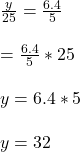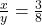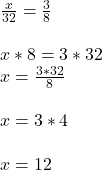## Find the value of x and y so that both proportions will be correct: x/y = 3/8 and y/25 = 6.4/5

Question

Find the value of x and y so that both proportions will be correct: x/y = 3/8 and y/25 = 6.4/5

in progress 0
6 months 2021-08-25T08:10:13+00:00 1 Answers 8 views 0